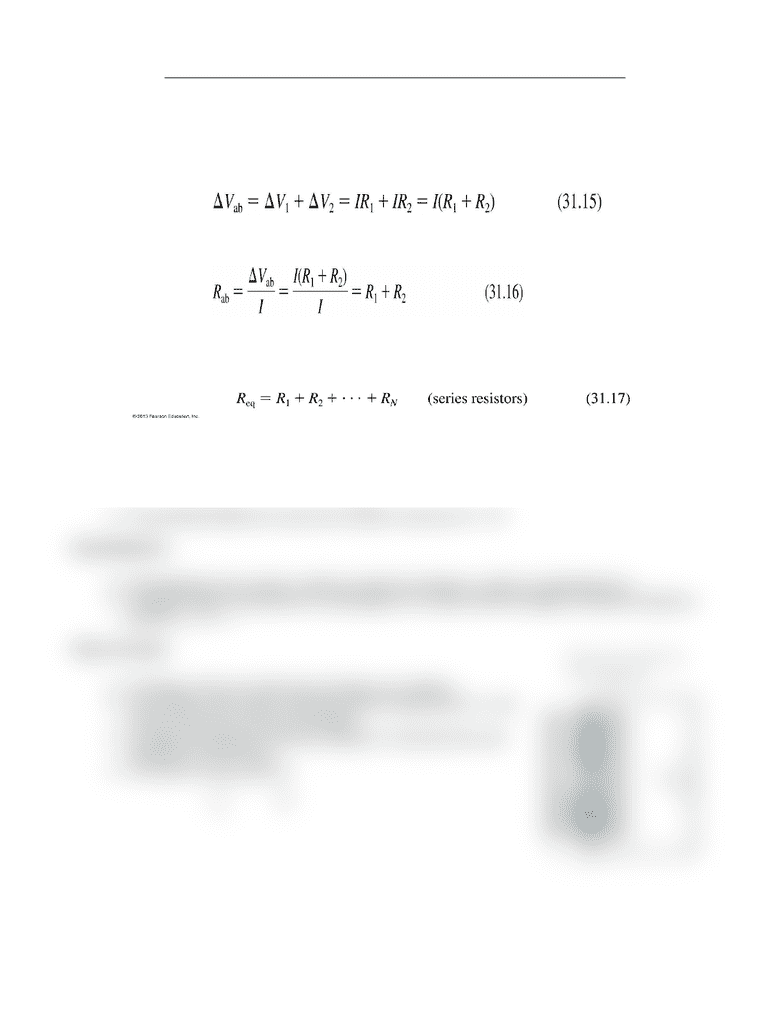# PHYS 142 Lecture Notes - Lecture 18: Internal Resistance, Ammeter

81 views1 pages
School
Department
CoursePHYS 142: Electromagnetism and Optics - Lecture 18: Circuits Part 2
Series Resistors:
The total potential difference between two resistors R1 and R2 connected in series is the sum
of the individual potential differences across R1 and R2
Suppose we replace R1 and R2 with a single resistor with the same current I and potential
difference ΔV.
Ohm’s law gives resistance between the two resistors:
Resistors that are aligned end to end, with no junctions between them, are called series
resistors or, sometimes, resistors “in series.”
The current I is the same through all resistors placed in series.
If we have N resistors in series, their equivalent resistance is:
Ammeters:
We can measure the current by breaking the connection and inserting an ammeter in series.
The resistance of the ammeter is negligible.
The potential difference across the resistor must be ΔVR = IR
Real Batteries:
Real batteries have what is called an internal resistance, which is symbolized by r.
A single resistor connected to a real battery is in series with the battery’s internal resistance,
giving Req = R + r.
Short Circuits:
The figure shows an ideal wire shorting out a battery.
If the battery were ideal, shorting it with an ideal wire (R = 0 Ω)
would cause the current to be infinite.
In reality, the battery’s internal resistance r becomes the only
resistance in the circuit.
The short-circuit current is:
Unlock document

This preview shows half of the first page of the document.
Unlock all 1 pages and 3 million more documents.

## Document Summary

Phys 142: electromagnetism and optics - lecture 18: circuits part 2. The total potential difference between two resistors r1 and r2 connected in series is the sum of the individual potential differences across r1 and r2. Suppose we replace r1 and r2 with a single resistor with the same current i and potential difference v. Ohm"s law gives resistance between the two resistors: Resistors that are aligned end to end, with no junctions between them, are called series resistors or, sometimes, resistors in series. The current i is the same through all resistors placed in series. If we have n resistors in series, their equivalent resistance is: We can measure the current by breaking the connection and inserting an ammeter in series. The resistance of the ammeter is negligible. The potential difference across the resistor must be vr = ir. Real batteries have what is called an internal resistance, which is symbolized by r.

# Get access

\$10 USD/m
Billed \$120 USD annually
Homework Help
Class Notes
Textbook Notes